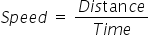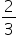Mathematics
Easy

Question

# Rebecca rides her horse with a constant speed of 15 miles per hour. Time taken by her to ride 10 miles is _____________ minutes.

## 10203040Hint:

## The correct answer is: 40

### The given value of speed is 15 miles per hourSpeed = 15 miles/hourNow, as the rate or speed is constant we can use this value to find the value of time required to travel 10 milesLet’s see the formulaRearranging the formula to find time. We will multiply both sides by time and divide by speed.We have to find time in minutes. So we will convert hour into minutes. 1 hour is 60 minutes. So we will multiply the above equation by 60.Time =× 60Time = 40 minutesTherefore, the time taken by Rebecca to ride the distance of 10 miles is 40 minutes.So the option which is ‘40’, is the right option

We have to be careful about units in such questions. Same quantities must have same units.

### Related Questions to study#### With Turito Foundation.#### Get an Expert Advice From Turito.